# Raster Resampling for Discrete and Continuous Data

## How do you decide what interpolation method to use?

### Resampling Techniques in GIS

When you go from a 5-meter cell size to 10-meter cell size, cell size will be different in the output raster grid. When converting raster data between different coordinate systems, cell-centers don’t match.

In both situations, a resampling approach must be taken to specify how the output grid will take shape. But it’s not always an easy choice which resampling method to use because there’s more than one way to recalculate cell values.

We’ll highlight which resampling technique it is appropriate to use in given scenarios. We’ll also touch on how we use these resampling methods in a GIS environment. There are four common ways to resample raster grids in GIS.

• Nearest Neighbor
• Bilinear
• Cubic Convolution
• Majority

### 1 Nearest Neighbor Resampling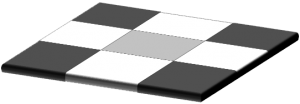The nearest neighbor technique doesn’t change any of the values from the input raster data set. It takes the cell center from the input raster data set to determine the closest cell center of the output raster. For processing speed, it’s generally the fastest because of its simplicity.

Because nearest neighbor resampling doesn’t alter any values in the output raster data set, it is ideal for categorical, nominal, and ordinal data.

When should you use nearest neighbor resampling?
Often, you use the nearest neighbor for discrete data like land cover classification, buildings, and soil types that have distinct boundaries and their limits are discrete.

When you resample this type of data, you should use the nearest neighbor resampling. For example, if you have a land cover classification raster grid, nearest neighbor will take the cell center value.

If agriculture has a discrete value of 7, the nearest neighbor method will never assign it a value of 7.2. It simply involves taking the output value from the nearest input layer cell center.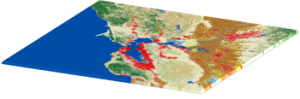### 2 Bilinear Interpolation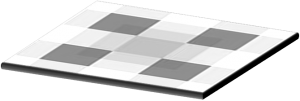Bilinear interpolation is a technique for calculating values of a grid location based on four nearby grid cells. It assigns the output cell value by taking the weighted average of the four neighboring cells in an image to generate new values.

It smooths the output raster grid, but not as much as cubic convolution. It’s useful when working with continuous data sets that don’t have distinct boundaries.

When should you use bilinear resampling?
Temperature gradients rasters, digital elevation models and annual precipitation grids are examples of when to use bilinear interpolation

For example, noise distance rasters don’t have discrete limits. In this case, this type of data varies continuously cell-to-cell to form a surface.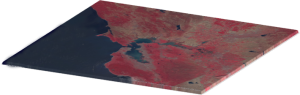### 3 Cubic Convolution Interpolation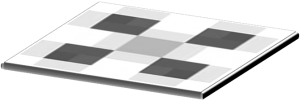Cubic convolution interpolation is similar to bilinear interpolation in that it takes the average of surrounding cells. Instead of using the four nearest cells, the output value is based on averaging the 16 nearest cells. As a result, processing time tends to increase for this method.

This method is generally used for continuous surfaces where much noise exists. Because it takes more neighboring cells compared to bilinear resampling, it’s good for smoothing data from the input raster grid.

When should you use cubic convolution interpolation?
Cubic convolution is ideal for noisy rasters like smoothing out a radar image or surface model.

Generally, we use cubic convolution much less than bilinear interpolation. In particular, it’s good for noise reduction. For example, a synthetic aperture radar image might benefit from cubic convolution interpolation technique because it reduces noise which is commonly seen in radar.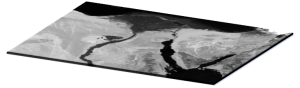### 4 Majority ResamplingWhile the nearest neighbor resampling takes the cell-center from the input raster data, the majority algorithm uses the most common values within the filter window.

Similar to the nearest neighbor algorithm, this technique is commonly used for discrete data like land cover classification and other types of raster grids with distinct boundaries.

When should you use majority resampling?
We often use majority filter for land cover so the most popular class remains in the output raster.

For example, if the filter window finds 3 cells of agriculture land cover and 2 cells of road, the output data set will be classified as agriculture. This is because the agriculture land cover class is the most popular cell within the filter window. When compared with nearest neighbor resampling, the resulting data set will often be smoother.### Raster Resampling: The Main Takeaway

Image processing has become more important to create images at different resolutions and coordinate system conversions. This is why we use image resampling techniques like the nearest neighbor, bilinear interpolation, cubic convolution, and majority interpolation.

In GIS, nearest neighbor resampling does not change any of the values of the output cells from the input raster dataset. This makes the nearest neighbor suitable for discrete data like land cover classification maps. While nearest neighbor resampling took the cell center from the input raster data set, majority resampling is based on the most common values found within the filter window.

The bilinear interpolation technique works best for continuous data. This is because output cells are calculated based on the relative position of the four nearest values from the input grid.

When you have even more noise in the input raster grid, this is when cubic convolution can be more advantageous. It smooths out the output grid because it takes the 16 nearest cells from the input data set.